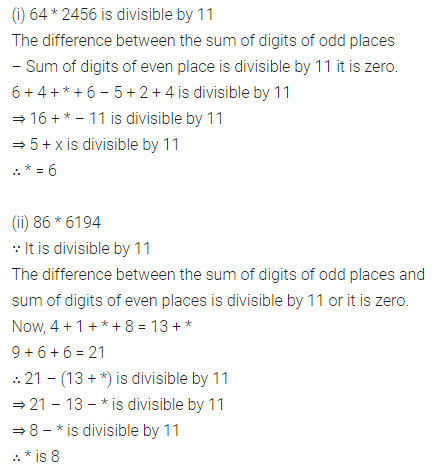## ML Aggarwal Class 8 Solutions for ICSE Maths Chapter 5 Playing with Numbers Ex 5.3

Question 1.
Which of the following numbers are divisible by 5 or by 10:
(i) 87035
(ii) 75060
(iii) 9685
(iv) 10730
Solution: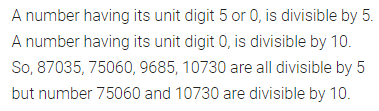Question 2.
Which of the following numbers are divisible by 2, 4 or 8:
(i) 67894
(ii) 5673244
(iii) 9685048
(iv) 6533142
(v) 75379
Solution:Question 3.
Which of the following numbers are divisible by 3 or 9:
(i) 45639
(ii) 301248
(iii) 567081
(iv) 345903
(v) 345046
Solution:Question 4.
Which of the following numbers are divisible by 11:
(i) 10835
(ii) 380237
(iii) 504670
(iv) 28248
Solution: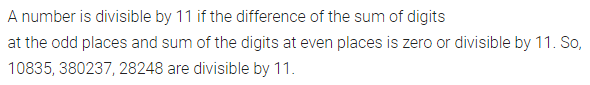Question 5.
Which of the following numbers are divisible by 6:
(i)15414
(ii) 213888
(iii) 469876
Solution:Question 6.
Which of the following numbers are divisible by 7:
(i) 46f8894875
(ii) 3794856
(iii) 39823
Solution: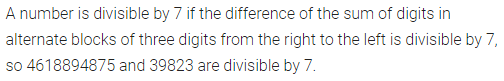Question 7.
(i) If 34x is a multiple of 3, where x is a digit, what is the value of x?
(ii) If 74×5284 is a multiple of 3, where x is a digit, find the value(s) of x.
Solution: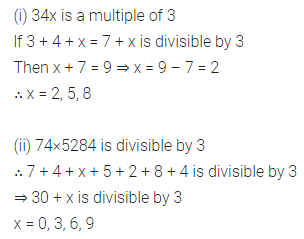Question 8.
If 42z3 is a multiple of 9, where z is a digit, what is the value of z?
Solution: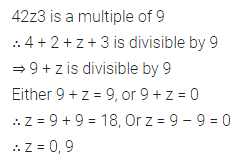Question 9.
In each of the following replace * by a digit so that the number formed is divisible by 9:
(i) 49 * 2207
(ii) 5938 * 623
Solution:Question 10.
In each of the following replace * by a digit so that the number formed is divisible by 6:
(i) 97 * 542
(ii) 709 * 94
Solution: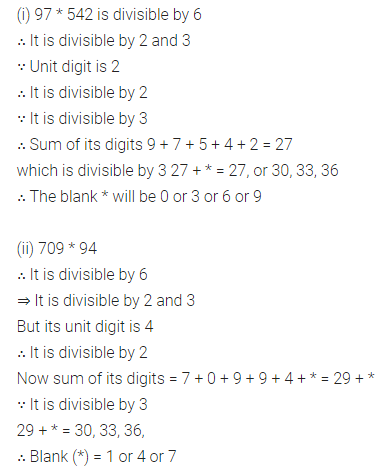Question 11.
In each of the following replace * by a digit so that the number formed is divisible by 11:
(i) 64*2456
(ii) 86*6194
Solution: